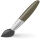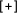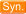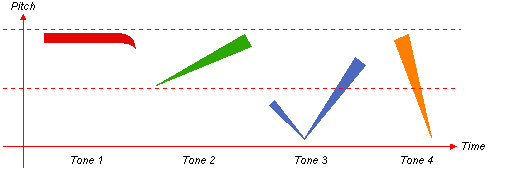"Remainder"  Chinese-English DictionaryDICTIONARY OPTIONS
CHARACTERS : Simplified Traditional
PHONETIC : Pinyin Bopomofo EFEO Wade-Giles Yale
 其余 qí yú the rest / the others / remaining / remainder / apart from them零 zero / nought / zero sign / fractional / fragmentary / odd (of numbers) / (placed between two numbers to indicate a smaller quantity followed by a larger one) / fraction / (in mathematics) remainder (after division) / extra / to wither and fall / to wither剩余 shèng yú remainder / surplus余额 yú é balance (of an account, bill etc) / surplus / remainder尾数 wěi shù remainder (after rounding a number) / decimal part (of number after the decimal point) / mantissa (i.e. fractional part of common logarithm in math.) / small change / balance (of an account)残渣 cán zhā remainder / filtered out residue / sediment / waste product / debris / detritus / rubbish零头 líng tóu odd / scrap / remainder余数 yú shù remainder (in division)尾 tail / remainder / remnant / extremity / sixth of the 28 constellations / classifier for fish馀数 yú shù (numerical) remainder余存 yú cún remainder / balance余留 yú liú remainder / fractional part (after the decimal point) / unfinished余项 yú xiàng remainder term (math) / remainder / residue余剩 yú shèng surplus / remainder整除 zhěng chú to divide exactly without remainder (in integer arithmetic) / exact division余 extra / surplus / remaining / remainder after division / (following numerical value) or more / in excess of (some number) / residue (math.) / after / I / me剩 to remain / to be left / to have as remainder馀 variant of 餘|余[yu2], remainder賸 have as remainder / trad. variant of 剩[sheng4]剩馀 shèng yú remainder / surplus中国剩余定理 Zhōng guó shèng yú dìng lǐ Chinese remainder theorem (math.)剩余定理 shèng yú dìng lǐ the Remainder Theorem孙子定理 Sūn zi dìng lǐ the Chinese remainder theorem料头 liào tóu remainder of cloth / scraps余数定理 yú shù dìng lǐ the Remainder Theorem余留无符号数 yú liú wú fú hào shù unsigned remainder (i.e. the remainder after rounding to the nearest integer)